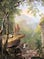Asher Brown Durand’s 𝘒𝘪𝘯𝘥𝘳𝘦𝘥 𝘚𝘱𝘪𝘳𝘪𝘵𝘴 (1849), a classic Transcendentalist painting that puts great emphasis on natural beauty and the meekness of man.

# The Right Philosophy for Our Times

## How Transcendentalism can revitalize our society’s love for the environment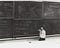Richard Feynman was a master of learning and the patience that deep understanding requires. His depth of knowledge was exactly what made him such a brilliant teacher. US Department of Energy.

# On Learning Deeply

A few years ago, I remember being faced with an introductory physics problem. It was the first time I had encountered the idea of acceleration, and I was puzzled by the notation for it.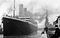Kaggle’s famous Titanic Dataset is a great place for people to begin their journey on applied machine learning.

# Naive Bayes Classification II: Application

## Applying the Bayes’ Rule to design a classifier in Python from scratch, and applying it on the Titanic Dataset

This article explains the probability theory that underlies the concept of Naive Bayes’, so if you’re looking for a theoretical understanding, see that.

I have a GitHub Repository of my homemade Naive Bayes Classifier here. It includes a submission to the Titanic Dataset. In my experiment, it actually scored 5% higher than the builtin Scikit-Learn Naive Bayes.

The nice thing about Naive Bayes’ is that the computations that underlie it are quite simple, as opposed to something like a neural network or even a support vector machine. We can create our own `MultinomialNaiveBayes()` class, which takes in a matix of…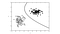Technically not the exact type of Naive Bayes Classification I was thinking. // astroML

# Naive Bayes Classification I: Theory

## How we can use elementary probability theory to find Bayes’ Theorem, and how we can use this to create a classifier

If you are looking for the practical implementation of a Naive-Bayes model from scratch, part II of this article explains that. I highly encourage you to read this first to understand the theory:

The rise of deep learning has to lead many of us to forget the importance (and often, superiority) of shallow learning algorithms like Naive Bayes.

Different learning algorithms use different branches of mathematics to arrive at a sensible conclusion on some set of data. Modern neural networks use a combination of linear algebra and multivariable calculus, archaic perceptrons used linear algebra and a simple addition/subtraction update rule…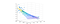In Recursive Least Squares, new points are appearing all the time and we need to adjust our plane to fit that. Multiple Linear Regression // Mathworks

# Recursive Least Squares

## Exploring Recursive Least Squares (RLS) and using the Sherman-Morrison-Woodbury Formula and Python

The mathematics here should be tackled with individuals who have completed an introductory linear algebra course. For those just looking for the code implementation, visit the GitHub repository here.

The Normal Equations for Least Squares are ubiquitous and for good reason. Apart from very large datasets, the Normal Equations are easy to use, easily generalizable to datasets with multiple variables, and easy to remember.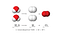Electrolysis of Water. JSquish // Wikimedia Commons

# Balancing Chemical Equations using Python

## Using Python and some basic linear algebra concepts, we can balance chemical equations

I’ll outline my theoretical approach to the problem in python here, using only some code, and you can get an idea of what is going on. I’ll link to the code on GitHub for the actual code.

Balancing chemical equations is a common activity in high-school classrooms and beyond. The question is (and is, for nearly any activity) — can we automate this process?

The answer is a bold yes, and there are a few ways that we can approach the problem. Mine might not be the fastest, but it is accurate for all chemical equations that do not include…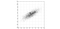PCA over a bivariate Gaussian distribution centered at (1,3). Image by Nicolás Guarín

# The Math of Principal Component Analysis (PCA)

## Using two different strategies rooted in linear algebra to understand the most important formula in dimensionality reduction

This article assumes the reader is comfortable with the contents covered in any introductory linear algebra course — orthogonality, eigendecompositions, spectral theorem, Singular Value Decomposition (SVD)…

Confusion of the proper method to do Principal Component Analysis (PCA) is almost inevitable. Different sources espouse different methods, and any learner quickly deduces that PCA isn’t really a specific algorithm, but a series of steps that may vary, with the final result being the same: data that is simplified into a more concise set of features.

After talking about the basic goal of PCA, I’ll explain the mathematics behind two commonly shown ways…

# Watch my Complete Lecture Series on Neural Network Mathematics

This one’s a little unusual of a post since I don’t have any stories, algorithms, math or philosophy to write about, but I spent the past week putting together a complete lecture on the mathematics that underlie neural networks.

It’s about 5 hours long, but there in the description there are timestamps to every subject covered in the video, which is in the twenties or thirties.

Here’s the syllabus of covered topics if you’d like.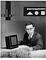Frank Rosenblatt’s beloved perceptron.

# The Rise and Fall of the Perceptron

## What is a perceptron, how the proto-neural network started (and stopped) interest in neural networks, the linear algebra behind them, and how group invariance theorem destroyed them.

If you were to gather a group of scientists from 1962 and ask them about their outlooks on the future and potential of artificial intelligence in solving computationally hard problems, the consensus would be generally positive.

If you were to ask the same group of scientists a decade later in 1972, the consensus would appear quite different, and contrary to the nature of scientific progress, it would be a lot more pessimistic.

We can attribute this change in attitude to the rise and fall of a single algorithm: the perceptron.

The perceptron algorithm, first proposed in 1958 by Frank Rosenblatt…

# The Normal Equation for Linear Regression

## The rationale and linear algebra behind Normal Equations, and the calculus as well.

This is a continuation of my Linear Algebra series, which should be viewed as an extra resource while going along with Gilbert Strang’s class 18.06 on OCW. This can be closely matched to Lecture 16 his series.

This article requires understanding of the four fundamental subspaces of a matrix, projection, projection of vectors onto planes, projection matrices, orthogonality, orthogonality of subspaces, elimination, transposes, and inverses. I would highly recommend understanding everything in Lecture 15.

In a previous article, I wrote about fitting a line to data points on a two-dimensional plane in the context of linear regression with gradient descent…## adam dhalla

15 y old learning about machine learning, as well as a lifelong naturalist. Climate activist in Vancouver. Writer. Visit me @ adamdhalla.com